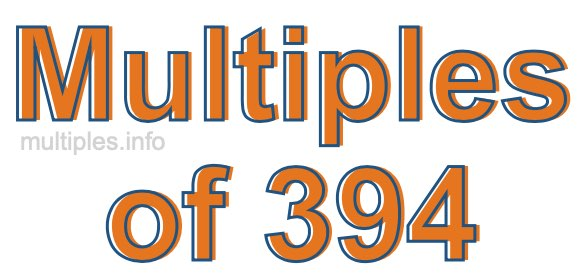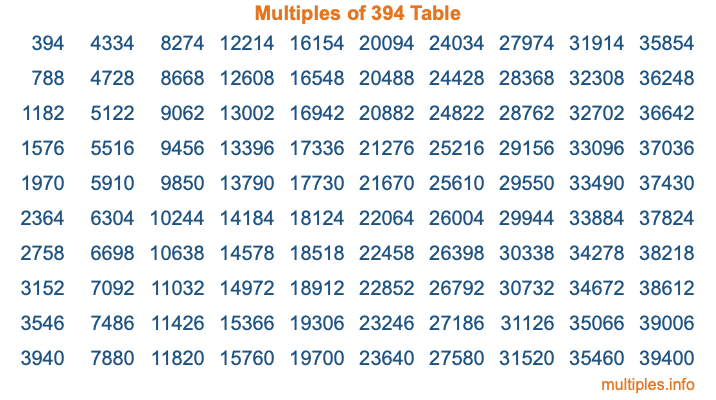Multiples of 394Welcome to the Multiples of 394 page. Here we will first teach you everything you will ever need to know about the multiples of 394, and then give you a study guide summary of everything we taught you to make sure you remember it all. Use this page to look up facts and learn information about the multiples of 394. This page will make you a multiples of three hundred ninety-four expert!

Definition of Multiples of 394
Multiples of 394 are all the numbers that when divided by 394 equal an integer. Each of the multiples of 394 are called a multiple. A multiple of 394 is created by multiplying 394 by an integer.

Therefore, to create a list of multiples of 394, you start with 1 multiplied by 394, then 2 multiplied by 394, then 3 multiplied by 394, and so on for as long as you want. Thus, the list of the first five multiples of 394 is 394, 788, 1182, 1576, and 1970. To see a larger list of multiples of 394, see the printable image of Multiples of 394 further down on this page. We also have a category where you can choose any nth multiple of 394.

Multiples of 394 Checker
The Multiples of 394 Checker below checks to see if any number of your choice is a multiple of 394. In other words, it checks to see if there is any number (integer) that when multiplied by 394 will equal your number. To do that, we divide your number by 394. If the the quotient is an integer, then your number is a multiple of 394.

Is  a multiple of 394?

Least Common Multiple of 394 and ...
A Least Common Multiple (LCM) is the lowest multiple that two or more numbers have in common. This is also called the smallest common multiple or lowest common multiple and is useful to know when you are adding our subtracting fractions. Enter one or more numbers below (394 is already entered) to find the LCM.

Check out our LCM Calculator if you need more details about the Least Common Multiple or if you need the LCM for different numbers for adding and subtraction fractions.

nth Multiple of 394
As we stated above, 394 is the first multiple of 394, 788 is the second multiple of 394, 1182 is the third multiple of 394, and so on. Enter a number below to find the nth multiple of 394.

th multiple of 394

Multiples of 394 vs Factors of 394
394 is a multiple of 394 and a factor of 394, but that is where the similarities end. All postive multiples of 394 are 394 or greater than 394. All positive factors of 394 are 394 or less than 394.

Below is the beginning list of multiples of 394 and the factors of 394 so you can compare:

Multiples of 394: 394, 788, 1182, 1576, 1970, etc.

Factors of 394: 1, 2, 197, 394

As you can see, the multiples of 394 are all the numbers that you can divide by 394 to get a whole number. The factors of 394, on the other hand, are all the whole numbers that you can multiply by another whole number to get 394.

It's also interesting to note that if a number (x) is a factor of 394, then 394 will also be a multiple of that number (x).

Multiples of 394 vs Divisors of 394
The divisors of 394 are all the integers that 394 can be divided by evenly. Below is a list of the divisors of 394.

Divisors of 394: 1, 2, 197, 394

The interesting thing to note here is that if you take any multiple of 394 and divide it by a divisor of 394, you will see that the quotient is an integer.

Multiples of 394 Table
Below is an image of the first 100 multiples of 394 in a table. The table is in chronological order, column by column. The first column has the first ten multiples of 394, the second column has the next ten multiples of 394, and so on.The Multiples of 394 Table is also referred to as the 394 Times Table or Times Table of 394. You are welcome to print out our table for your studies.

Negative Multiples of 394
Although not often discussed or needed in math, it is worth mentioning that you can make a list of negative multiples of 394 by multiplying 394 by -1, then by -2, then by -3, and so on, to get the following list of negative multiples of 394:

-394, -788, -1182, -1576, -1970, etc.

Multiples of 394 Summary
Below is a summary of important Multiples of 394 facts that we have discussed on this page. To retain the knowledge on this page, we recommend that you read through the summary and explain to yourself or a study partner why they hold true.

There are an infinite number of multiples of 394.

A multiple of 394 divided by 394 will equal a whole number.

394 divided by a factor of 394 equals a divisor of 394.

The nth multiple of 394 is n times 394.

The largest factor of 394 is equal to the first positive multiple of 394.

394 is a multiple of every factor of 394.

394 is a multiple of 394.

A multiple of 394 divided by a divisor of 394 equals an integer.

394 divided by a divisor of 394 equals a factor of 394.

Any integer times 394 will equal a multiple of 394.

Multiples of a Number
Here you can get the multiples of another number, all with the same attention to detail as we did for multiples of 394 on this page.

Multiples of
Multiples of 395
Did you find our page about multiples of three hundred ninety-four educational? Do you want more knowledge? Check out the multiples of the next number on our list!